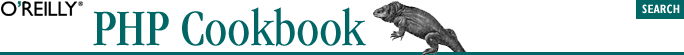home | O'Reilly's CD bookshelfs | FreeBSD | Linux | Cisco | Cisco Exam3.17. Program: Calendar

The pc_calendar() function shown in Example 3-4 prints out a month's calendar, similar to the Unix cal program. Here's how you can use the function:

// print the calendar for the current month
list(\$month,\$year) = explode(',',date('m,Y'));
pc_calendar(\$month,\$year);

The pc_calendar( ) function prints out a table with a month's calendar in it. It provides links to the previous and next month and highlights the current day.

Example 3-4. pc_calendar( )

<?php
function pc_calendar(\$month,\$year,\$opts = '') {
// set default options //
if (! is_array(\$opts)) { \$opts = array(); }
if (! isset(\$opts['today_color'])) { \$opts['today_color'] = '#FFFF00'; }
'<a href="'.\$_SERVER['PHP_SELF'].'?month=%d&year=%d">%s</a>';
}

list(\$this_month,\$this_year,\$this_day) = split(',',strftime('%m,%Y,%d'));
\$day_highlight = ((\$this_month == \$month) && (\$this_year == \$year));

list(\$prev_month,\$prev_year) =
split(',',strftime('%m,%Y',mktime(0,0,0,\$month-1,1,\$year)));

list(\$next_month,\$next_year) =
split(',',strftime('%m,%Y',mktime(0,0,0,\$month+1,1,\$year)));

?>
<tr>
<td align="left">
</td>
<td colspan="5" align="center">
<?php print strftime('%B %Y',mktime(0,0,0,\$month,1,\$year)); ?>
</td>
<td align="right">
</td>
</tr>
<?php
\$totaldays = date('t',mktime(0,0,0,\$month,1,\$year));

// print out days of the week
print '<tr>';
\$weekdays = array('Su','Mo','Tu','We','Th','Fr','Sa');
while (list(\$k,\$v) = each(\$weekdays)) {
print '<td align="center">'.\$v.'</td>';
}
print '</tr><tr>';
// align the first day of the month with the right week day
\$day_offset = date("w",mktime(0, 0, 0, \$month, 1, \$year));
if (\$day_offset > 0) {
for (\$i = 0; \$i < \$day_offset; \$i++) { print '<td>&nbsp;</td>'; }
}
\$yesterday = time() - 86400;

// print out the days
for (\$day = 1; \$day <= \$totaldays; \$day++) {
\$day_secs = mktime(0,0,0,\$month,\$day,\$year);
if (\$day_secs >= \$yesterday) {
if (\$day_highlight && (\$day == \$this_day)) {
print sprintf('<td align="center" bgcolor="%s">%d</td>',
\$opts['today_color'],\$day);
} else {
print sprintf('<td align="center">%d</td>',\$day);
}
} else {
print sprintf('<td align="center">%d</td>',\$day);
}
\$day_offset++;

// start a new row each week //
if (\$day_offset == 7) {
\$day_offset = 0;
print "</tr>\n";
if (\$day < \$totaldays) { print '<tr>'; }
}
}
// fill in the last week with blanks //
if (\$day_offset > 0) { \$day_offset = 7 - \$day_offset; }
if (\$day_offset > 0) {
for (\$i = 0; \$i < \$day_offset; \$i++) { print '<td>&nbsp;</td>'; }
}
print '</tr></table>';
}
?>

The pc_calendar( ) function begins by checking options passed to it in \$opts. The color that the current day is highlighted with can be passed as an RGB value in \$opts['today_color']. This defaults to #FFFF00, bright yellow. Also, you can pass a printf( )-style format string in \$opts['month_link'] to change how the links to the previous and next months are printed.

Next, the function sets \$day_highlight to true if the month and year for the calendar match the current month and year. The links to the previous month and next month are put into \$prev_month_link and \$next_month_link using the format string in \$opts['month_link'].

pc_calendar( ) then prints out the top of the HTML table that contains the calendar and a table row of weekday abbreviations. Using the day of the week returned from strftime('%w'), blank table cells are printed so the first day of the month is aligned with the appropriate day of the week. For example, if the first day of the month is a Tuesday, two blank cells have to be printed to occupy the slots under Sunday and Monday in the first row of the table.

After this preliminary information has been printed, pc_calendar( ) loops through all the days in the month. It prints a plain table cell for most days, but a table cell with a different background color for the current day. When \$day_offset reaches 7, a week has completed, and a new table row needs to start.